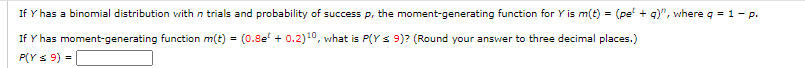# Question Solved1 AnswerIf Y has a binomial distribution with n trials and probability of success p, the moment-generating function for Y is m(t) = (pet + q)n, where q = 1 − p. If Y has moment-generating function m(t) = (0.8et + 0.2)10, what is P(Y ≤ 9)? (Round your answer to three decimal places.) P(Y ≤ 9) = 9)", where a = 1 - P. If Y has a binomial distribution with n trials and probability of success p, the moment-generating function for Y is m(t) = (pe! If Y has moment-generating function m(t) = (0.8e' +0.2), what is PCY S 9)? (Round your answer to three decimal places.) P(Y 9) =RHTUQL The Asker · Probability and Statistics

If Y has a binomial distribution with n trials and probability of success p, the moment-generating function for Y is m(t) = (pet + q)n, where q = 1 − p. If Y has moment-generating function m(t) = (0.8et + 0.2)10, what is P(Y ≤ 9)? (Round your answer to three decimal places.) P(Y ≤ 9) =Transcribed Image Text: 9)", where a = 1 - P. If Y has a binomial distribution with n trials and probability of success p, the moment-generating function for Y is m(t) = (pe! If Y has moment-generating function m(t) = (0.8e' +0.2), what is PCY S 9)? (Round your answer to three decimal places.) P(Y 9) =
More
Transcribed Image Text: 9)", where a = 1 - P. If Y has a binomial distribution with n trials and probability of success p, the moment-generating function for Y is m(t) = (pe! If Y has moment-generating function m(t) = (0.8e' +0.2), what is PCY S 9)? (Round your answer to three decimal places.) P(Y 9) =